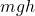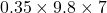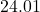## What is the gravitational potential energy of a soccer ball that is coming down if it has a mass of 0.35 kg and is coming down from a

Question

What is the gravitational potential energy of a soccer ball that is coming down if it
has a mass of 0.35 kg and is coming down from a height of 7 meters

in progress 0
3 weeks 2021-08-23T23:00:44+00:00 1 Answers 0 views 0

1. Here’s the solution,

gravitational potential energy :

=》where,

m = mass

g = acceleration due to gravity

h = height

So,

=》=》So, potential energy = 24.01 joules